# RD Sharma Solutions for Class 9 Maths Chapter 16 Circles Exercise 16.4

The RD Sharma Class 9 solutions for the chapter “Circle” exercise 16.4 is given here. In this exercise, students will learn about arcs and angles subtended by them. All the answers solved by subject experts using step-by-step problem solving approach which can help students understand this topic in a better and fun way.

## Download PDF of RD Sharma Solutions for Class 9 Maths Chapter 16 Circles Exercise 16.4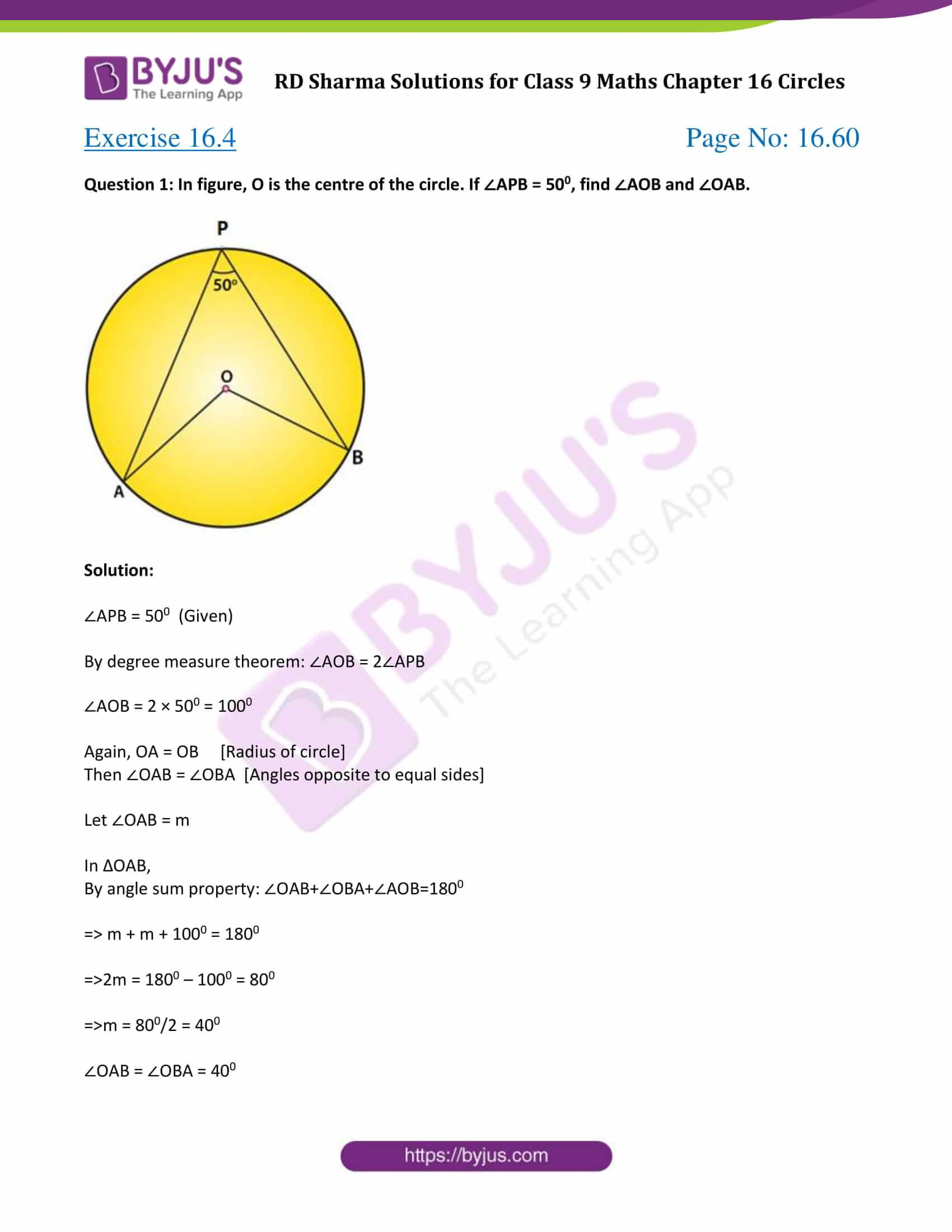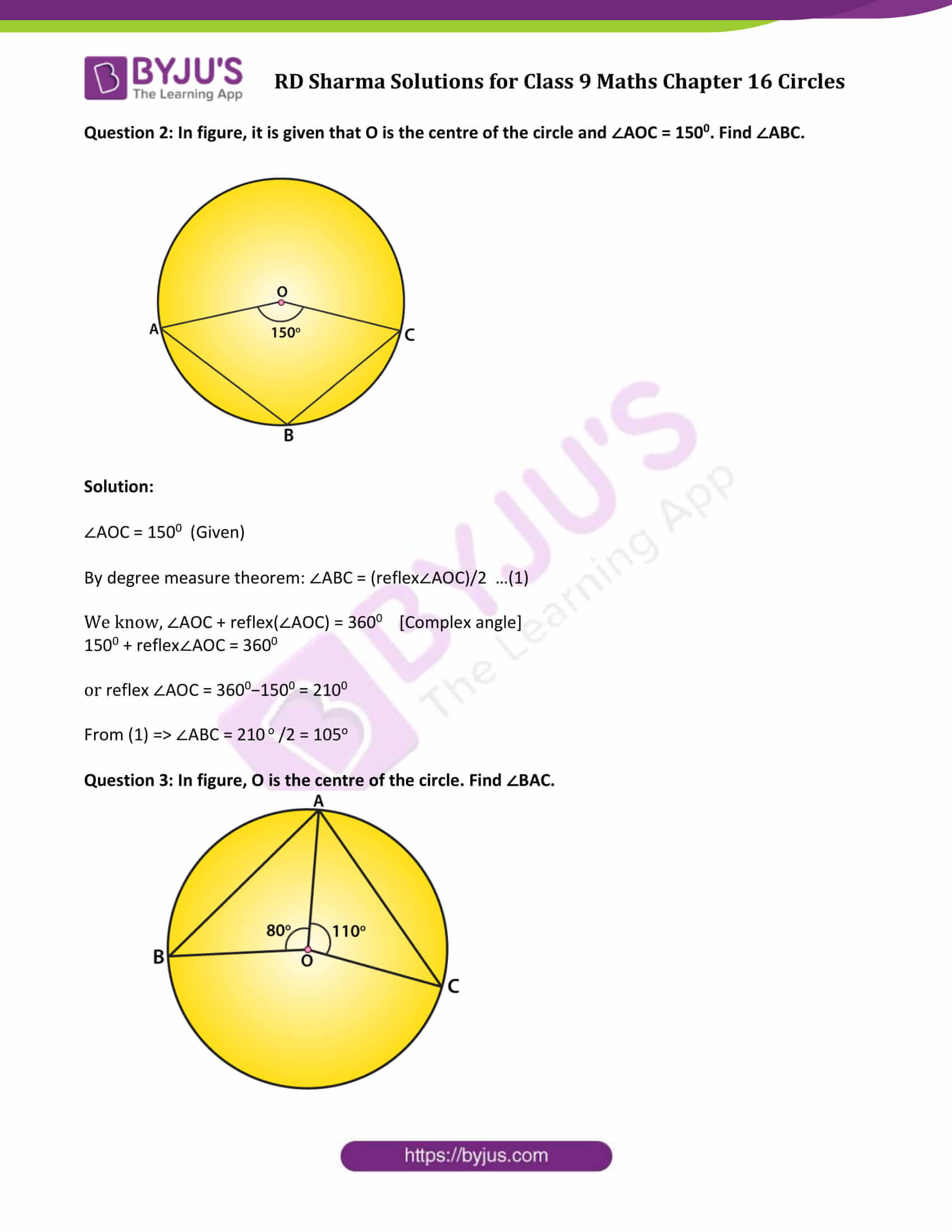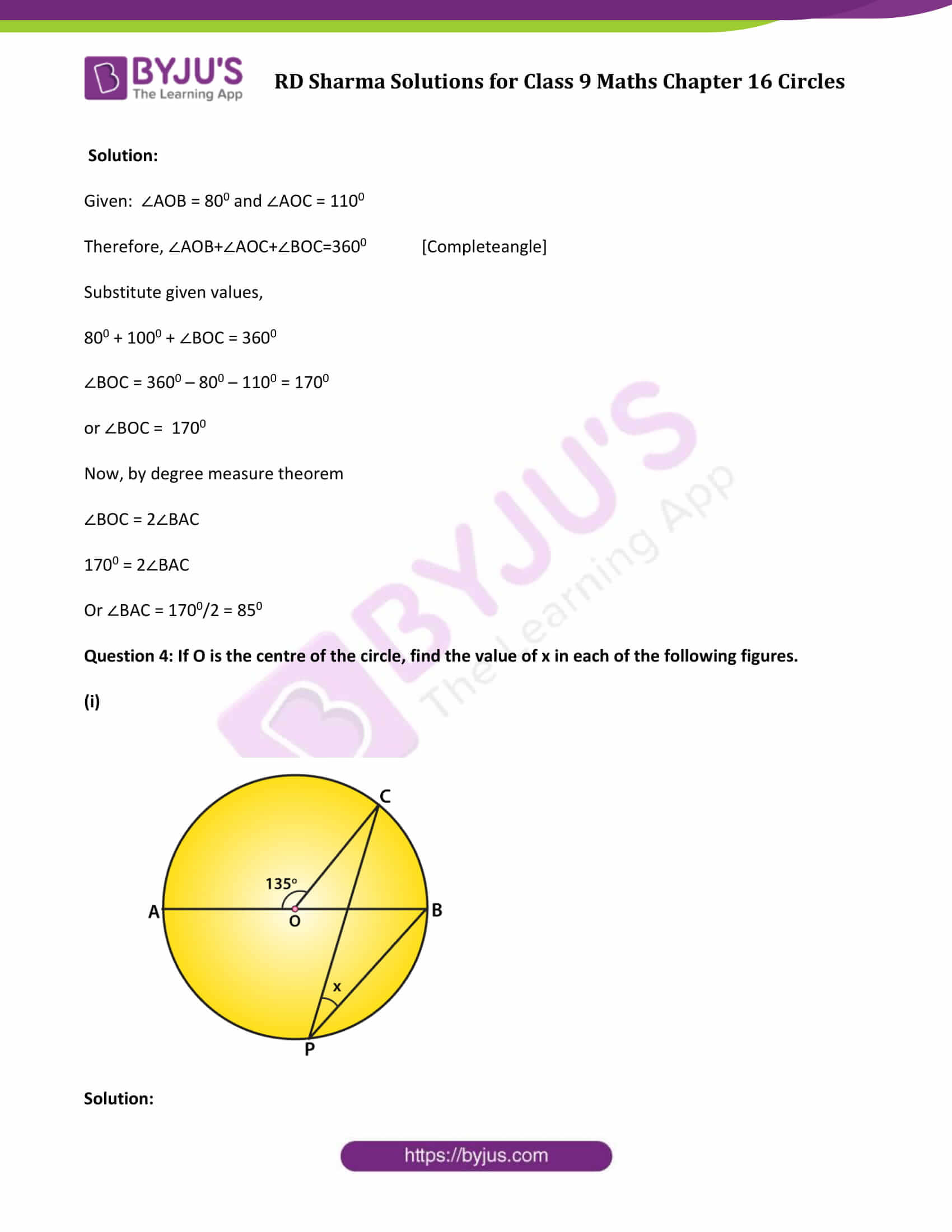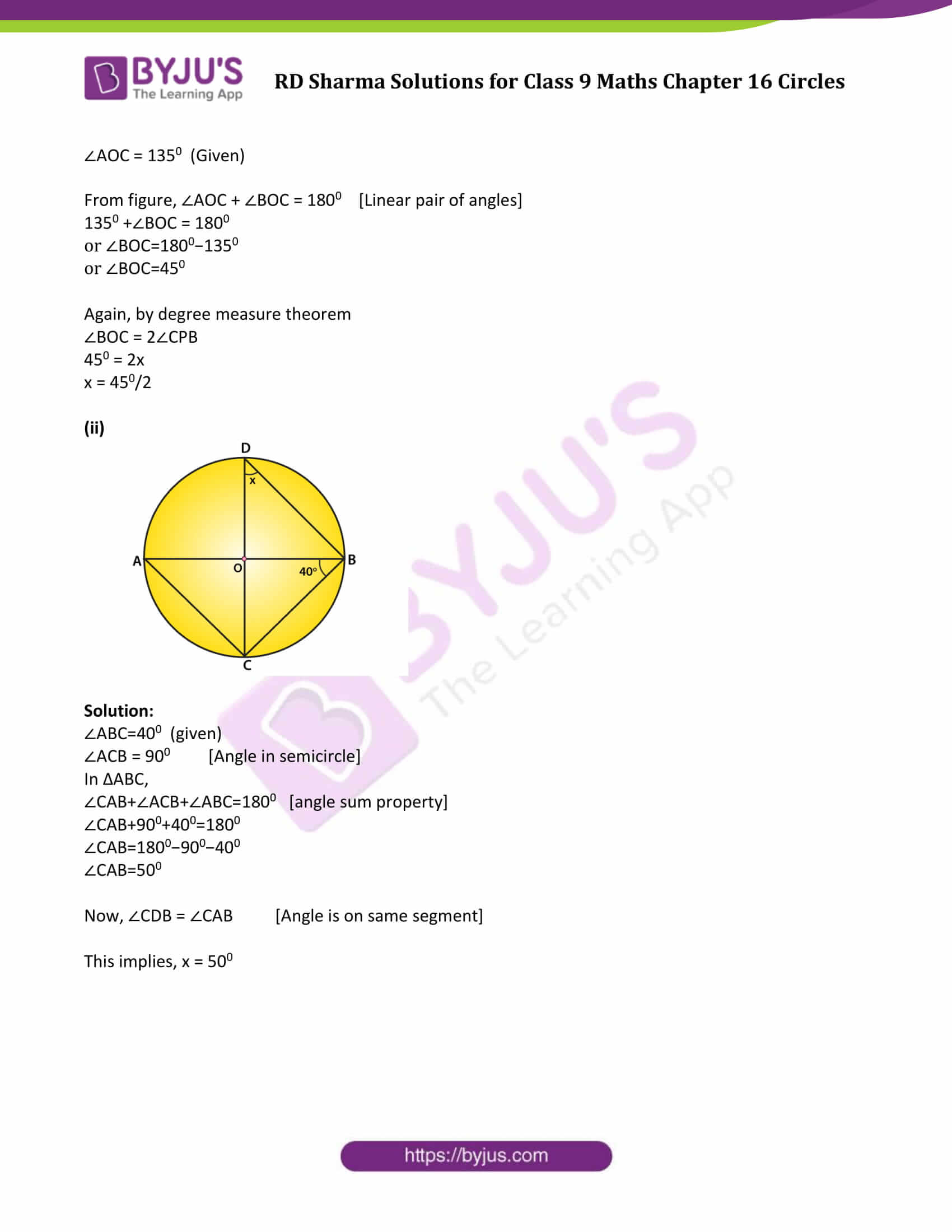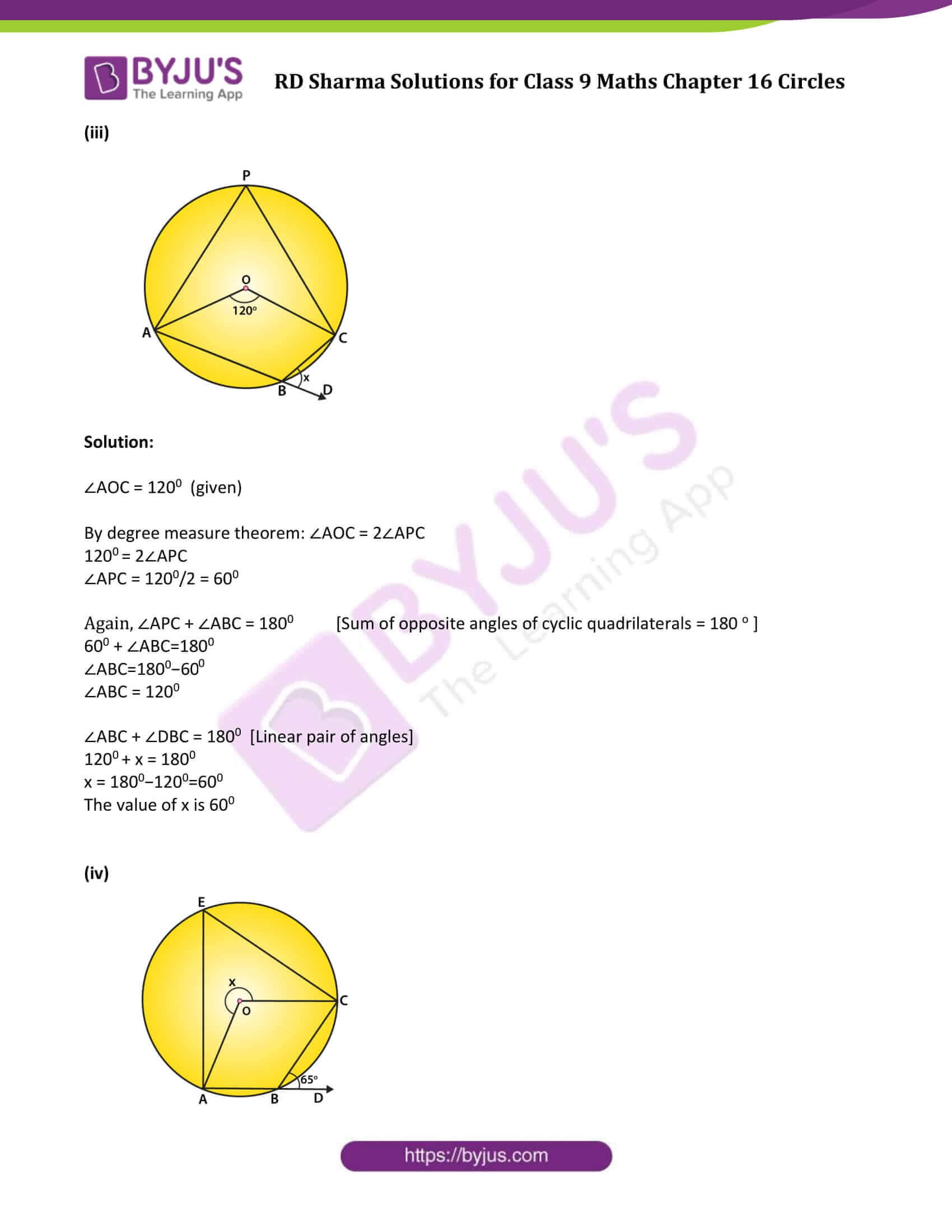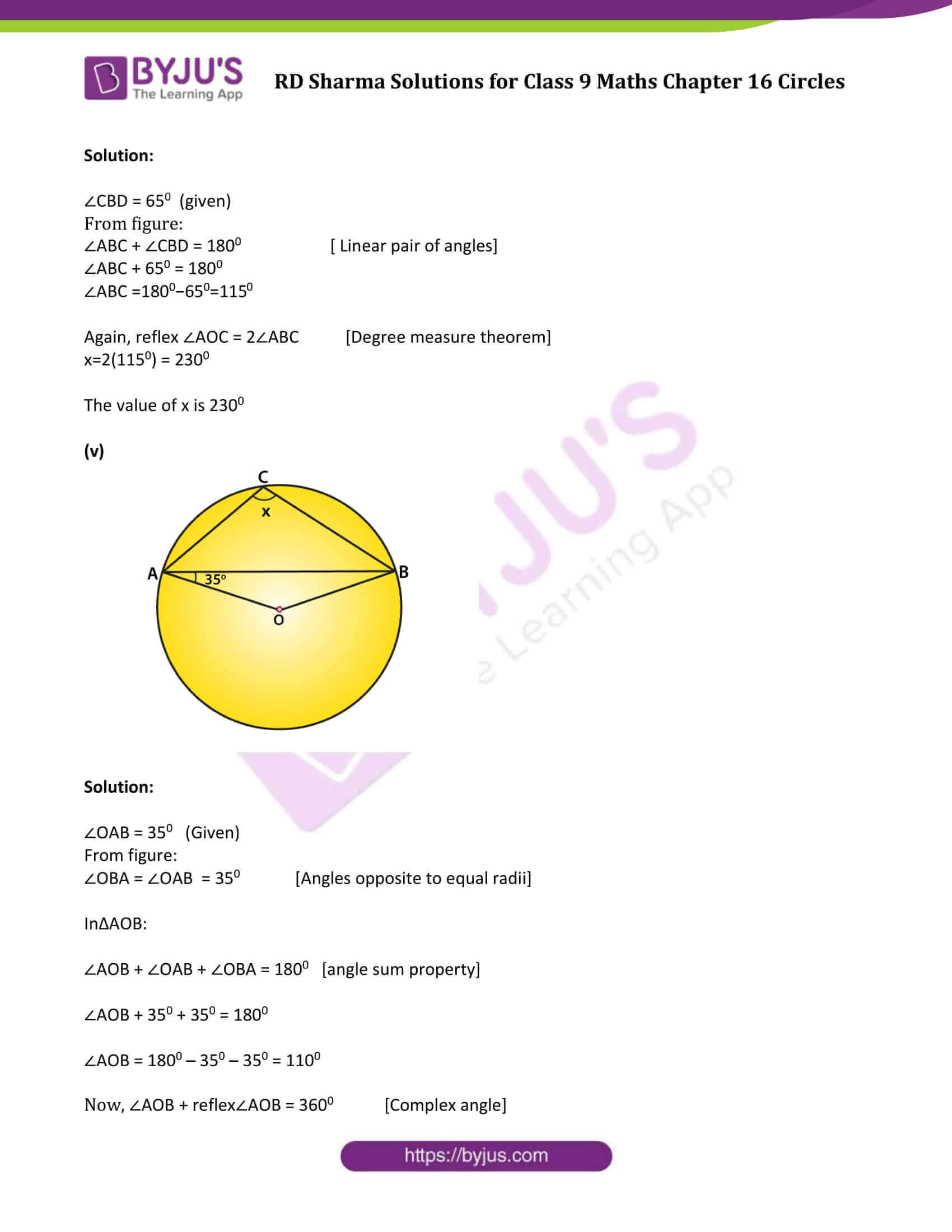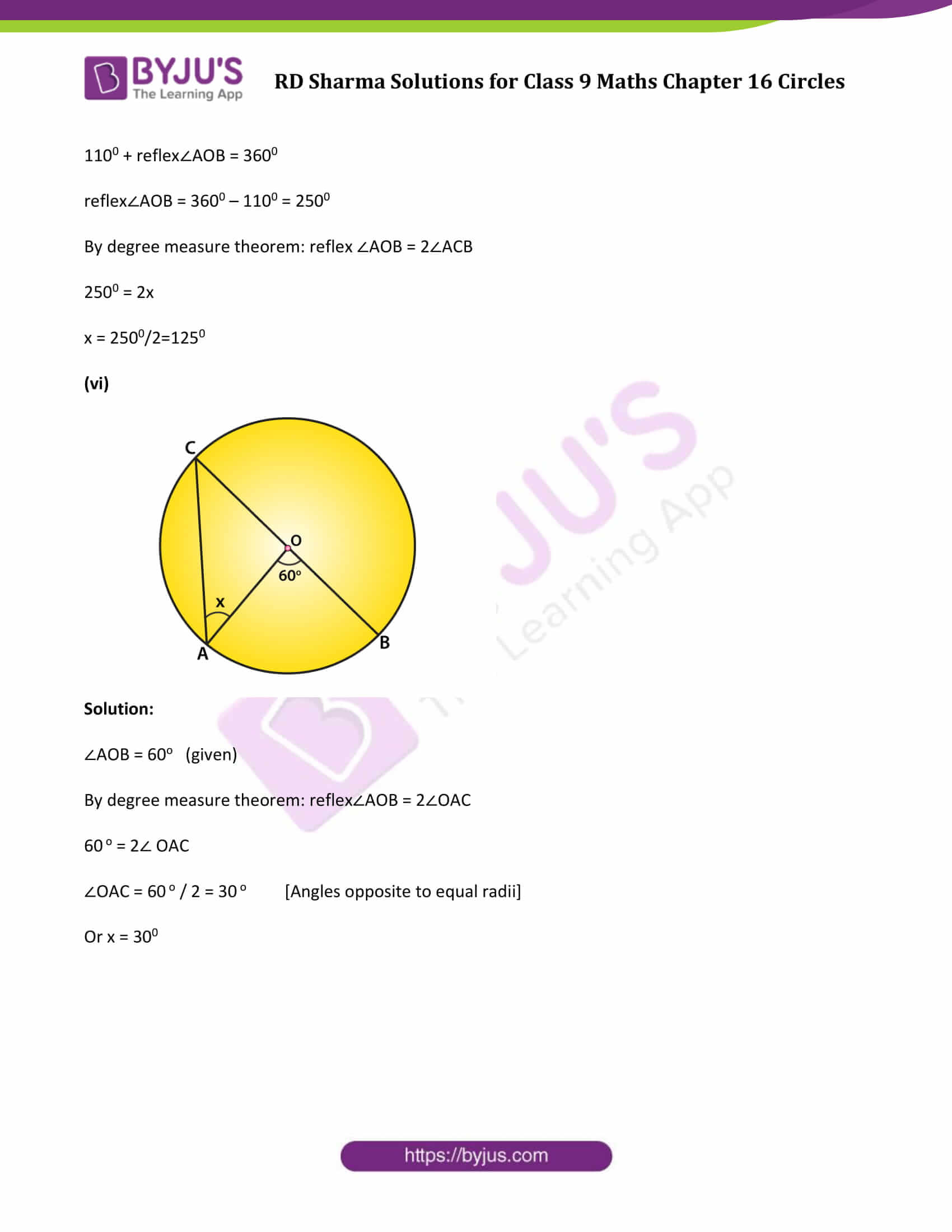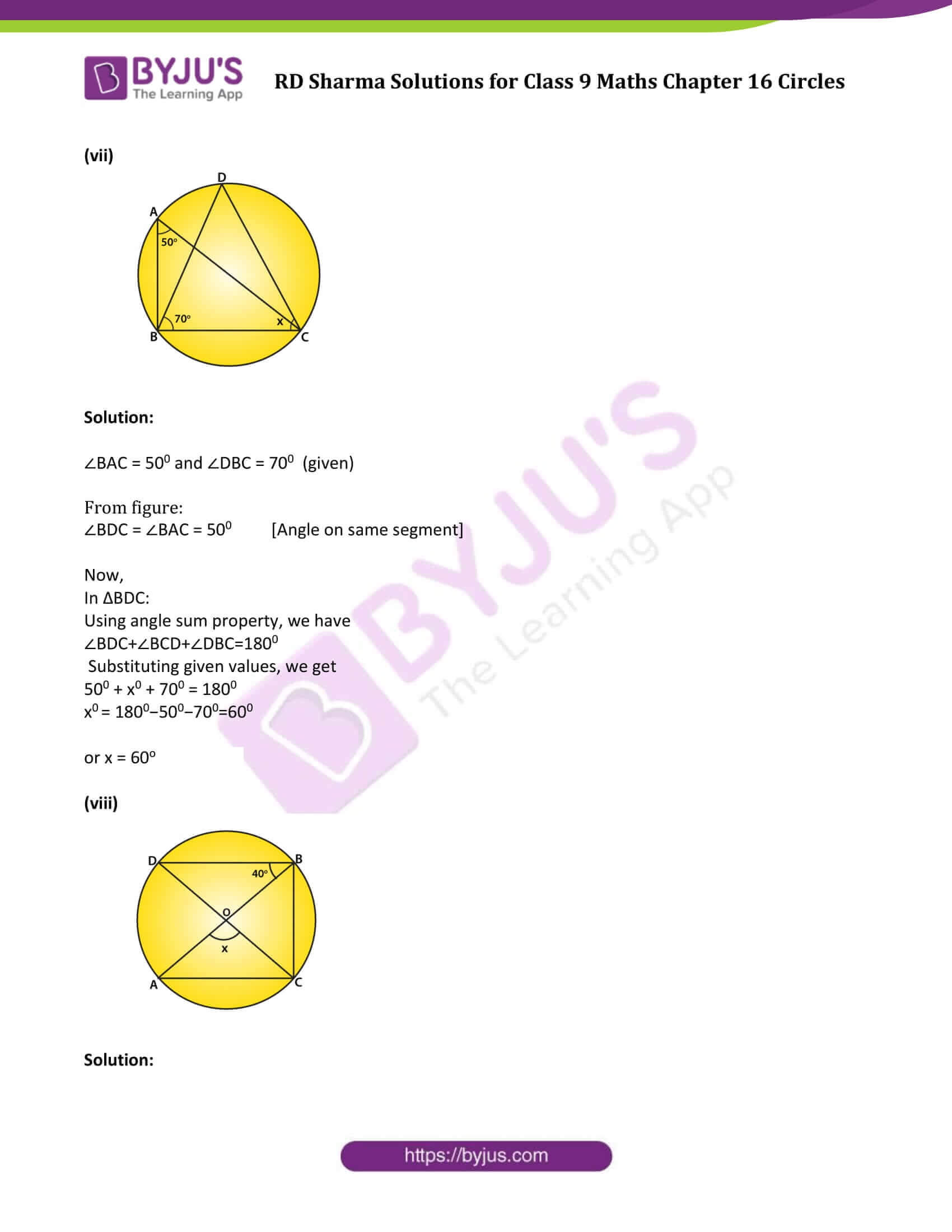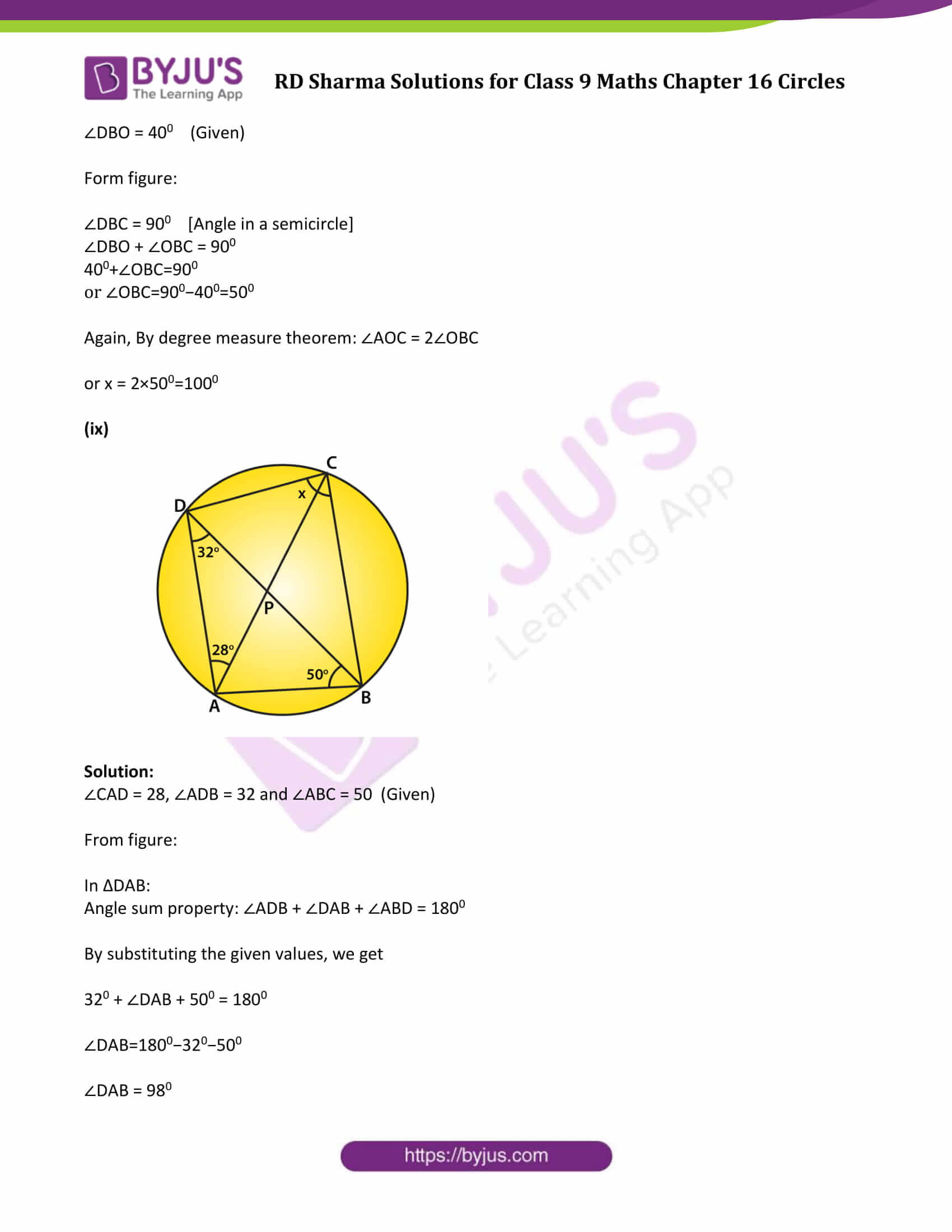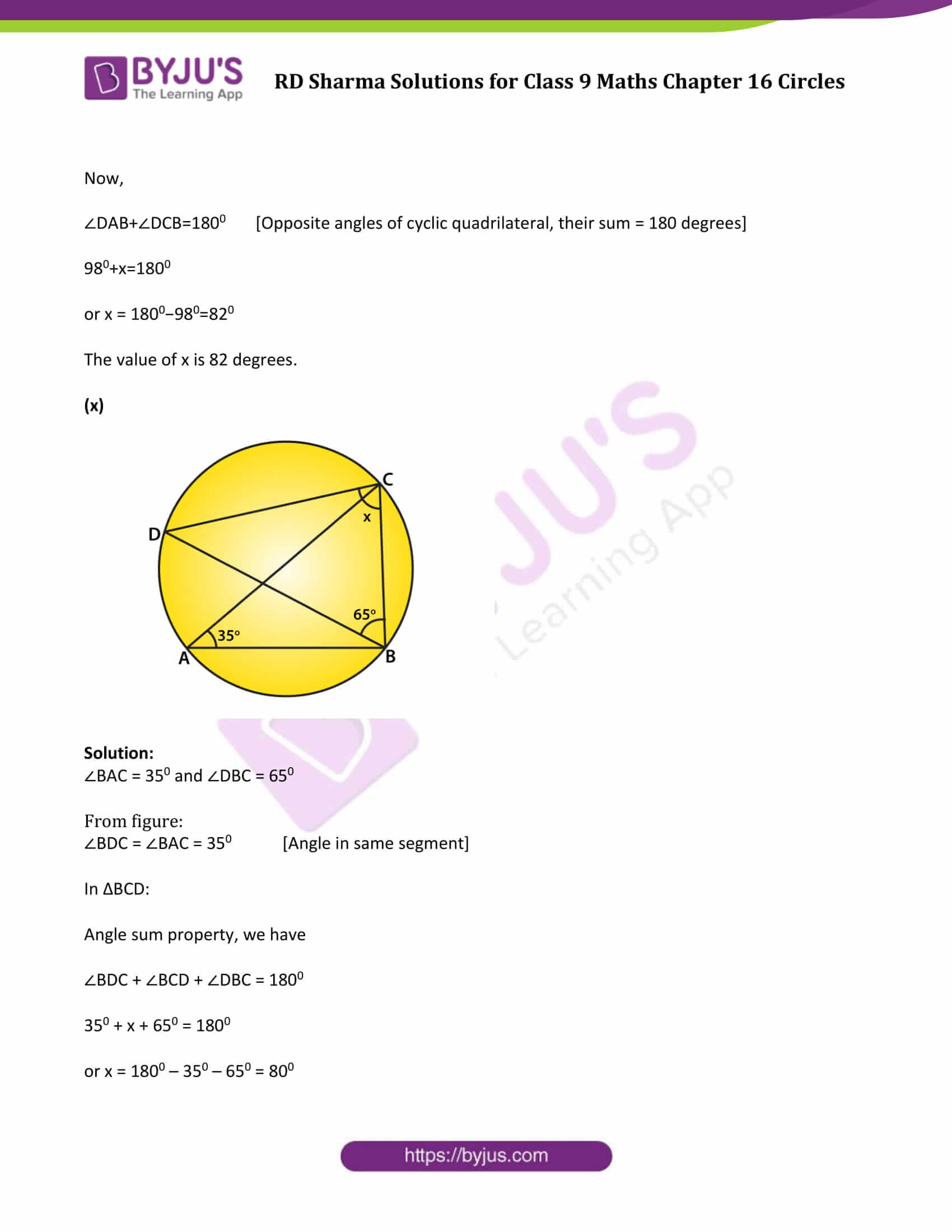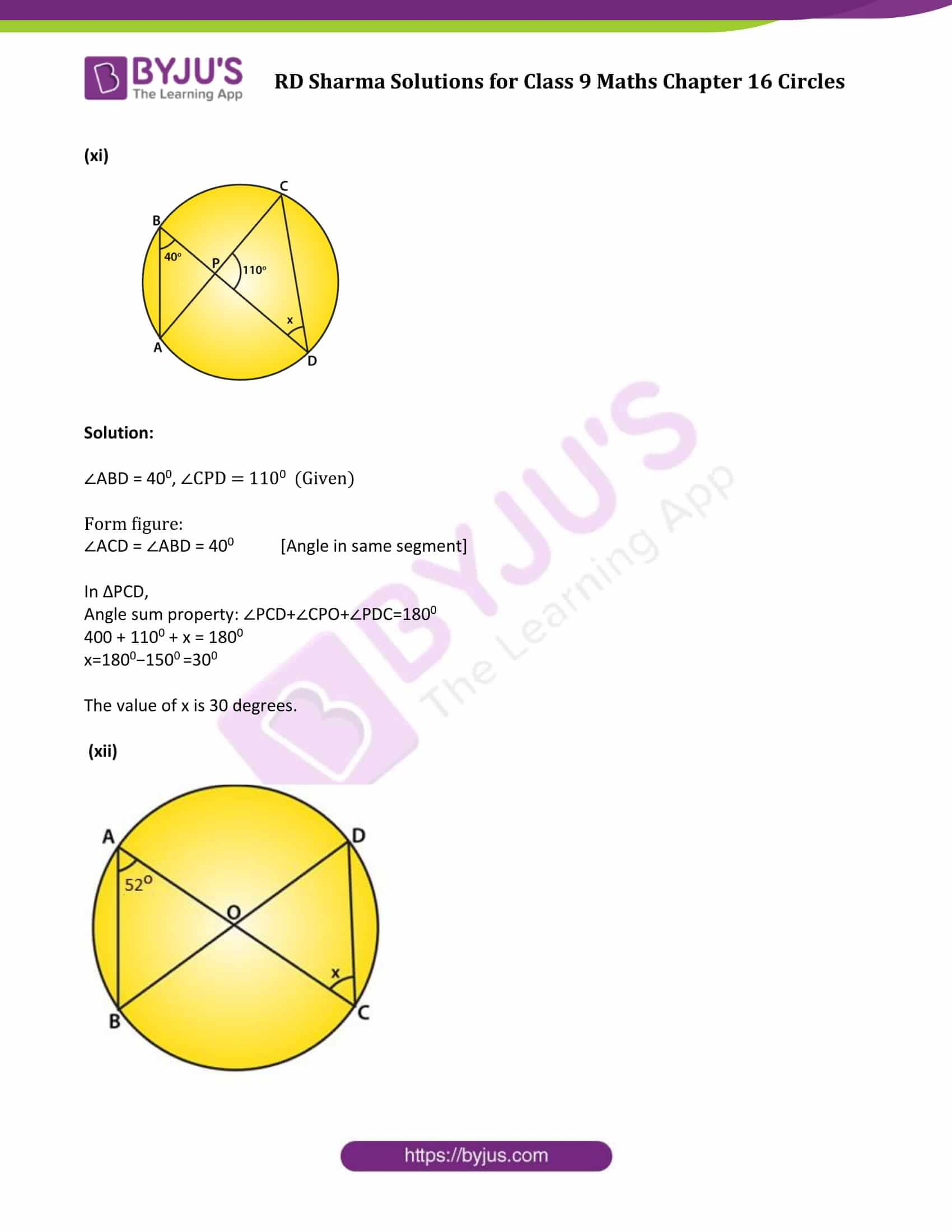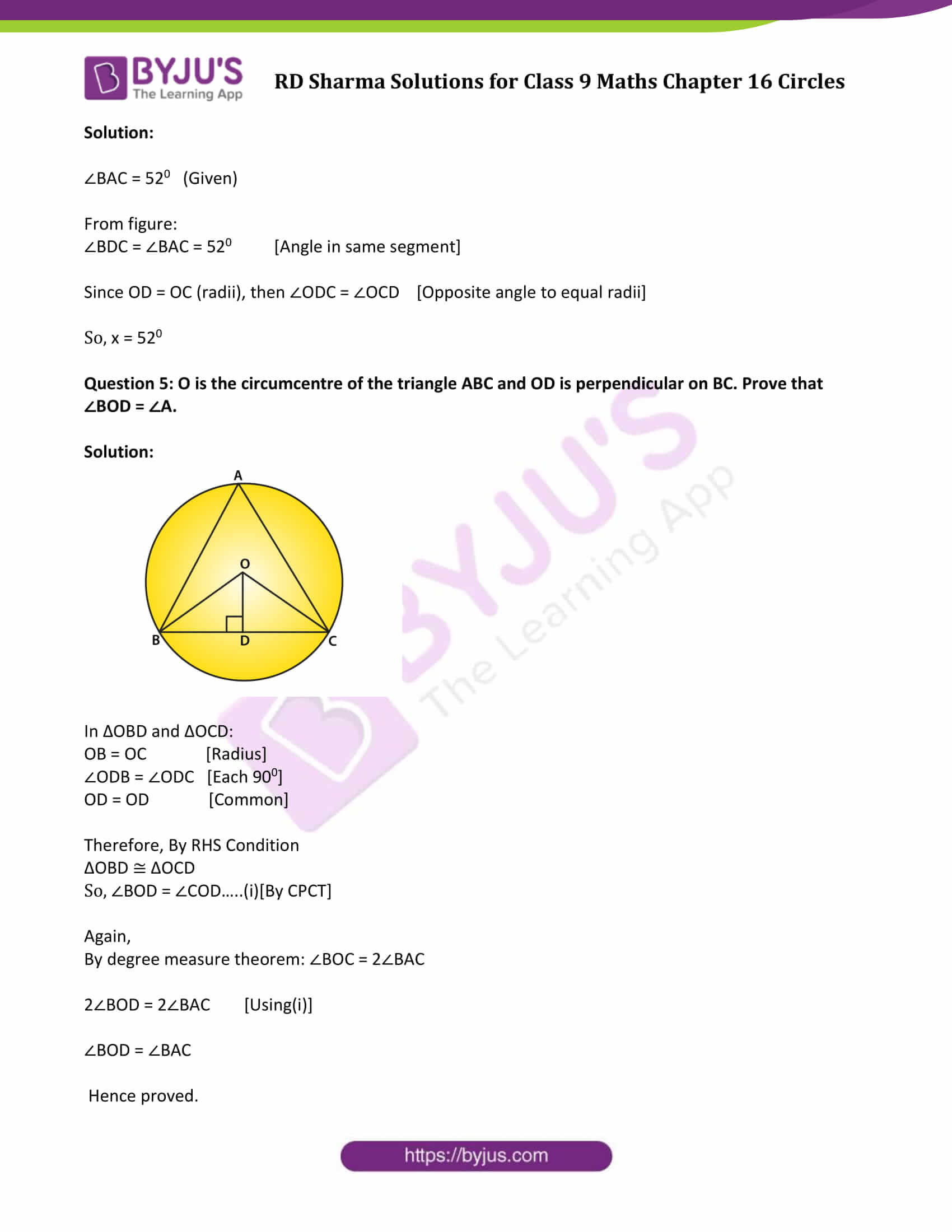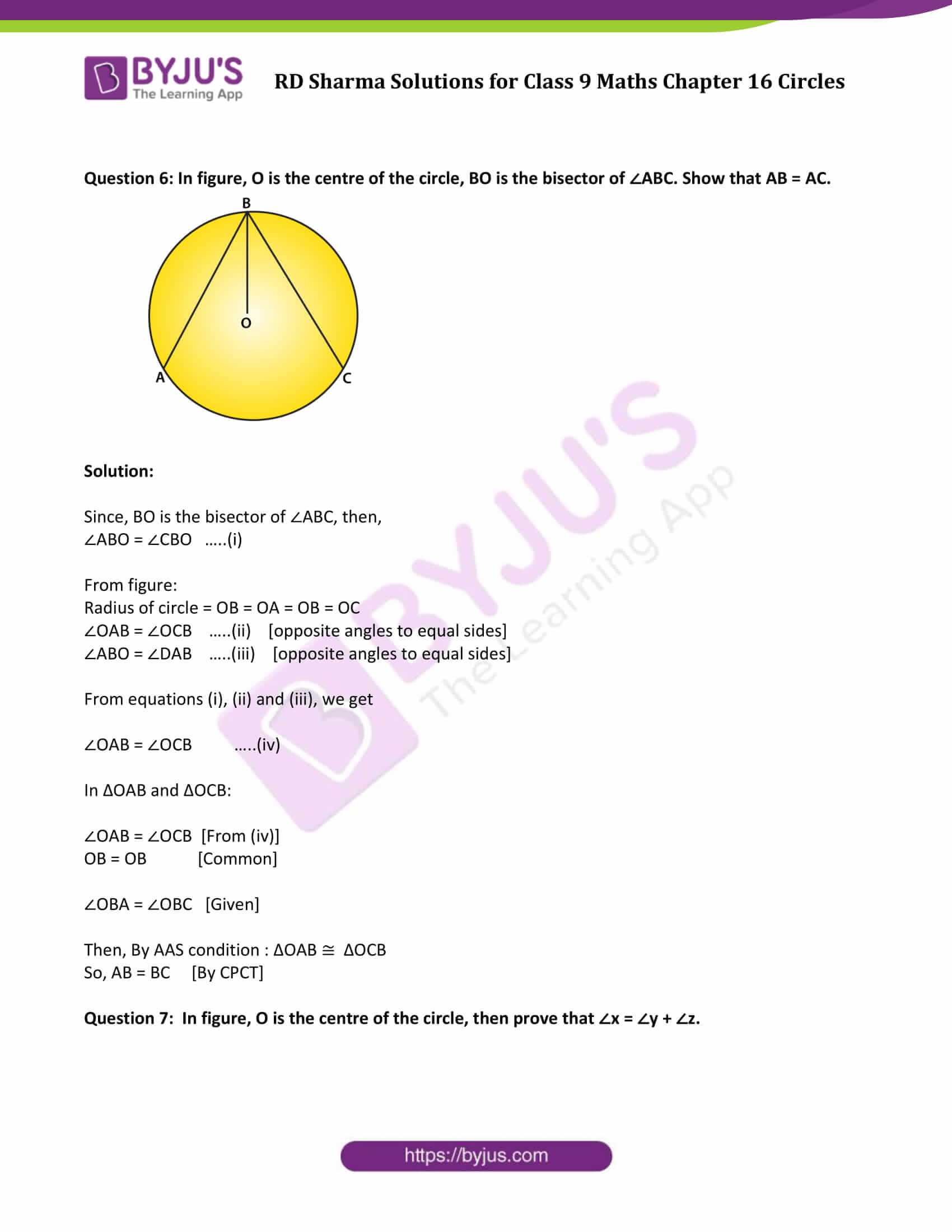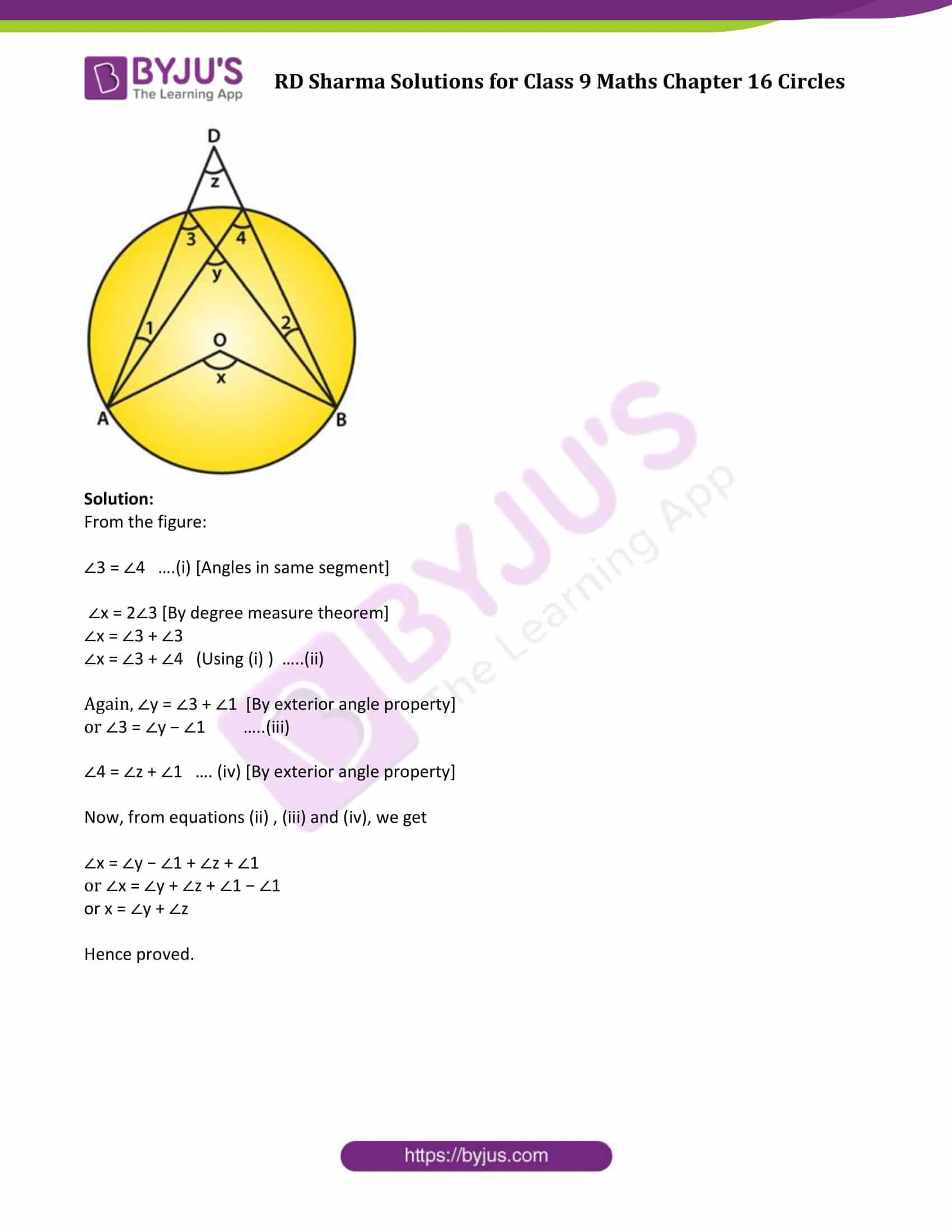### Access Answers to Maths RD Sharma Solutions for Class 9 Chapter 16 Circles Exercise 16.4 Page number 16.60

Question 1: In figure, O is the centre of the circle. If ∠APB = 500, find ∠AOB and ∠OAB.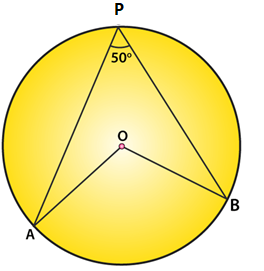Solution:

∠APB = 500 (Given)

By degree measure theorem: ∠AOB = 2∠APB

∠AOB = 2 × 500 = 1000

Again, OA = OB [Radius of circle]

Then ∠OAB = ∠OBA [Angles opposite to equal sides]

Let ∠OAB = m

In ΔOAB,

By angle sum property: ∠OAB+∠OBA+∠AOB=1800

=> m + m + 1000 = 1800

=>2m = 1800 – 1000 = 800

=>m = 800/2 = 400

∠OAB = ∠OBA = 400

Question 2: In figure, it is given that O is the centre of the circle and ∠AOC = 1500. Find ∠ABC.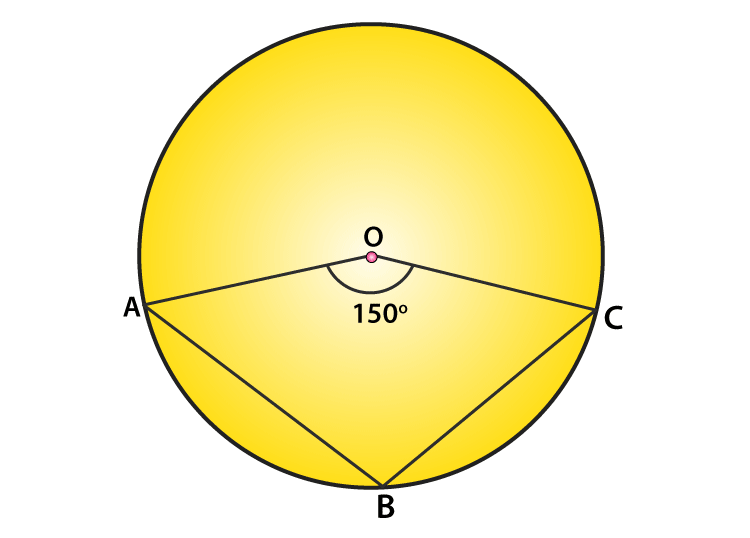Solution:

∠AOC = 1500 (Given)

By degree measure theorem: ∠ABC = (reflex∠AOC)/2 …(1)

We know, ∠AOC + reflex(∠AOC) = 3600 [Complex angle]

1500 + reflex∠AOC = 3600

or reflex ∠AOC = 3600−1500 = 2100

From (1) => ∠ABC = 210 o /2 = 105o

Question 3: In figure, O is the centre of the circle. Find ∠BAC.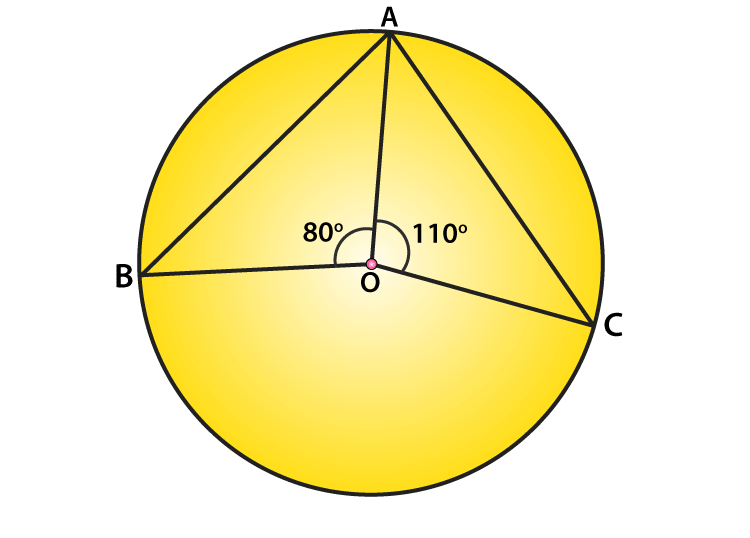Solution:

Given: ∠AOB = 800 and ∠AOC = 1100

Therefore, ∠AOB+∠AOC+∠BOC=3600 [Completeangle]

Substitute given values,

800 + 1000 + ∠BOC = 3600

∠BOC = 3600 – 800 – 1100 = 1700

or ∠BOC = 1700

Now, by degree measure theorem

∠BOC = 2∠BAC

1700 = 2∠BAC

Or ∠BAC = 1700/2 = 850

Question 4: If O is the centre of the circle, find the value of x in each of the following figures.

(i)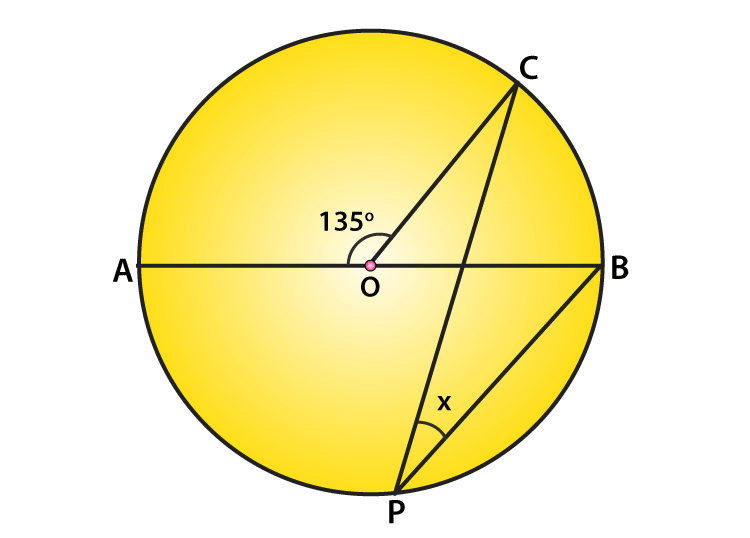Solution:

∠AOC = 1350 (Given)

From figure, ∠AOC + ∠BOC = 1800 [Linear pair of angles]

1350 +∠BOC = 1800

or ∠BOC=1800−1350

or ∠BOC=450

Again, by degree measure theorem

∠BOC = 2∠CPB

450 = 2x

x = 450/2

(ii)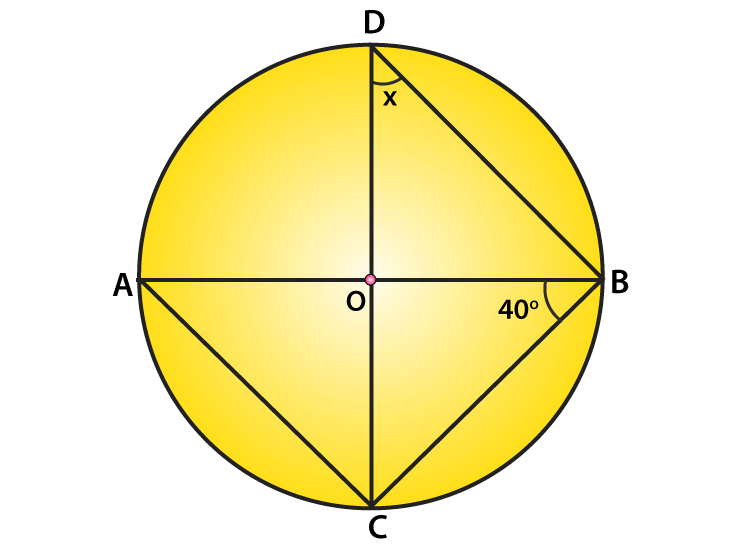Solution:

∠ABC=400 (given)

∠ACB = 900 [Angle in semicircle]

In ΔABC,

∠CAB+∠ACB+∠ABC=1800 [angle sum property]

∠CAB+900+400=1800

∠CAB=1800−900−400

∠CAB=500

Now, ∠CDB = ∠CAB [Angle is on same segment]

This implies, x = 500

(iii)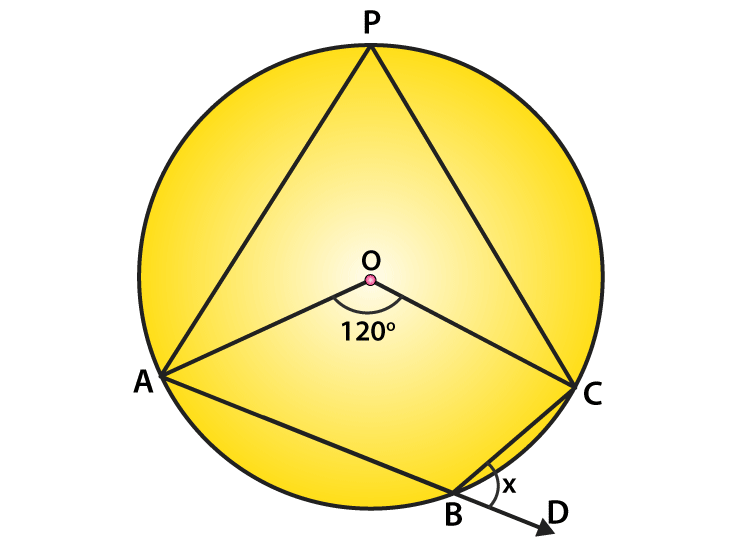Solution:

∠AOC = 1200 (given)

By degree measure theorem: ∠AOC = 2∠APC

1200 = 2∠APC

∠APC = 1200/2 = 600

Again, ∠APC + ∠ABC = 1800 [Sum of opposite angles of cyclic quadrilaterals = 180 o ]

600 + ∠ABC=1800

∠ABC=1800−600

∠ABC = 1200

∠ABC + ∠DBC = 1800 [Linear pair of angles]

1200 + x = 1800

x = 1800−1200=600

The value of x is 600

(iv)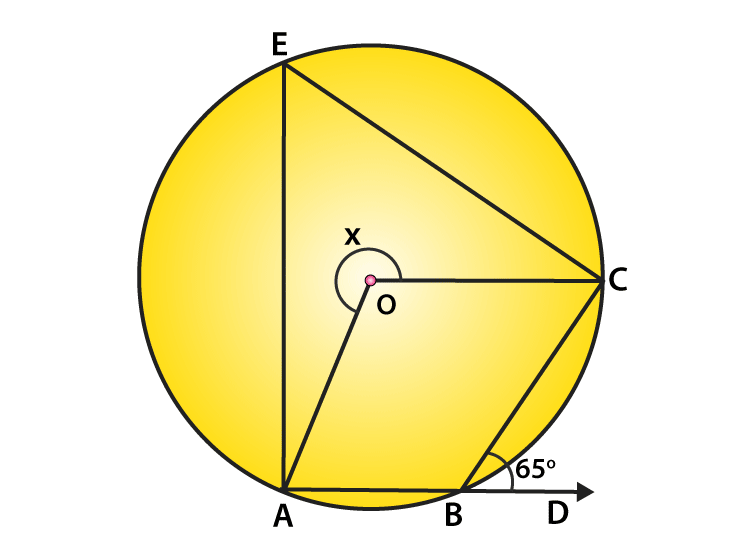Solution:

∠CBD = 650 (given)

From figure:

∠ABC + ∠CBD = 1800 [ Linear pair of angles]

∠ABC + 650 = 1800

∠ABC =1800−650=1150

Again, reflex ∠AOC = 2∠ABC [Degree measure theorem]

x=2(1150) = 2300

The value of x is 2300

(v)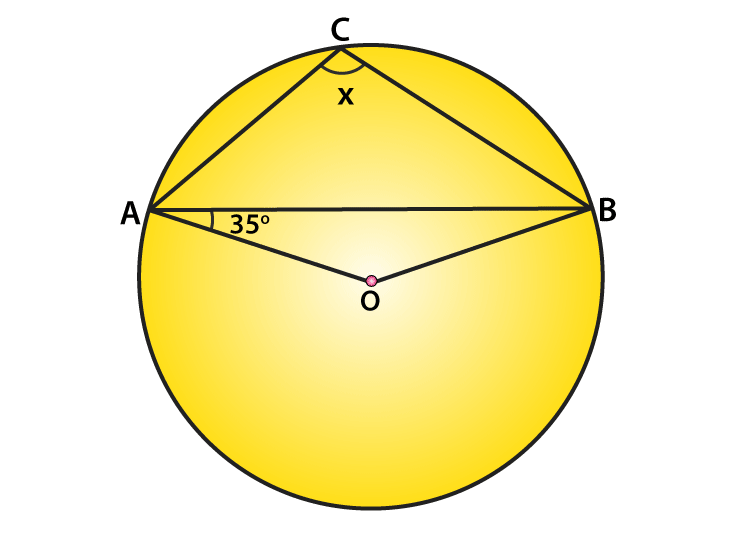Solution:

∠OAB = 350 (Given)

From figure:

∠OBA = ∠OAB = 350 [Angles opposite to equal radii]

InΔAOB:

∠AOB + ∠OAB + ∠OBA = 1800 [angle sum property]

∠AOB + 350 + 350 = 1800

∠AOB = 1800 – 350 – 350 = 1100

Now, ∠AOB + reflex∠AOB = 3600 [Complex angle]

1100 + reflex∠AOB = 3600

reflex∠AOB = 3600 – 1100 = 2500

By degree measure theorem: reflex ∠AOB = 2∠ACB

2500 = 2x

x = 2500/2=1250

(vi)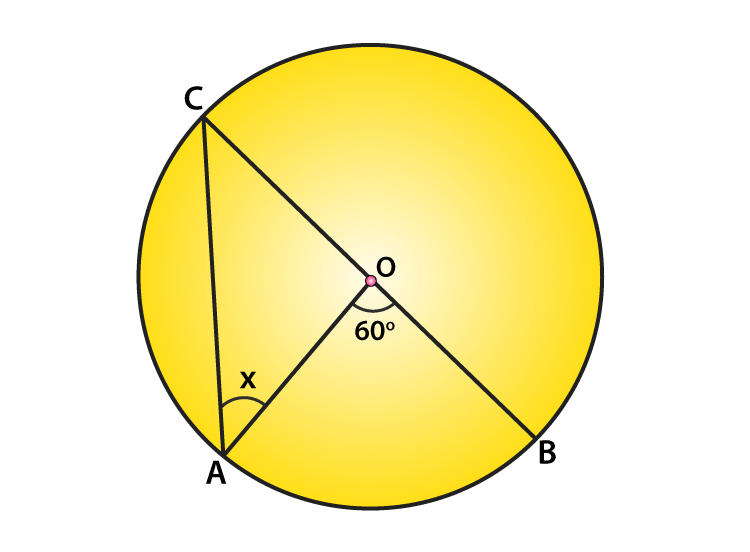Solution:

∠AOB = 60o (given)

By degree measure theorem: reflex∠AOB = 2∠OAC

60 o = 2∠ OAC

∠OAC = 60 o / 2 = 30 o [Angles opposite to equal radii]

Or x = 300

(vii)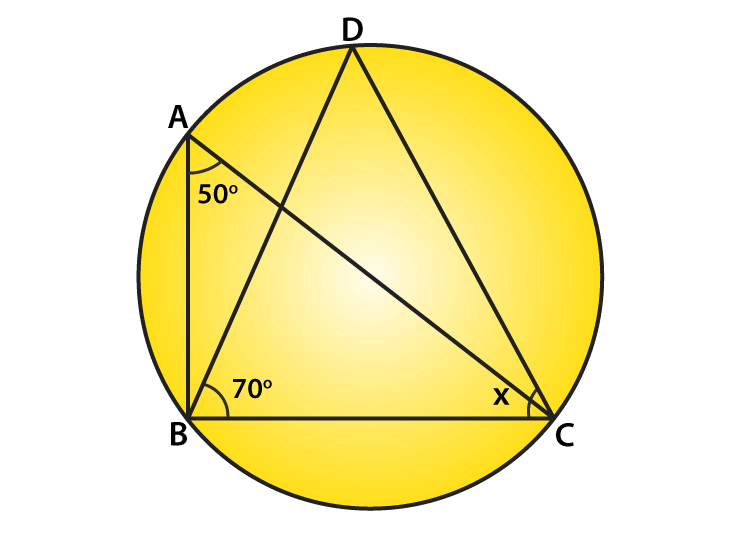Solution:

∠BAC = 500 and ∠DBC = 700 (given)

From figure:

∠BDC = ∠BAC = 500 [Angle on same segment]

Now,

In ΔBDC:

Using angle sum property, we have

∠BDC+∠BCD+∠DBC=1800

Substituting given values, we get

500 + x0 + 700 = 1800

x0 = 1800−500−700=600

or x = 60o

(viii)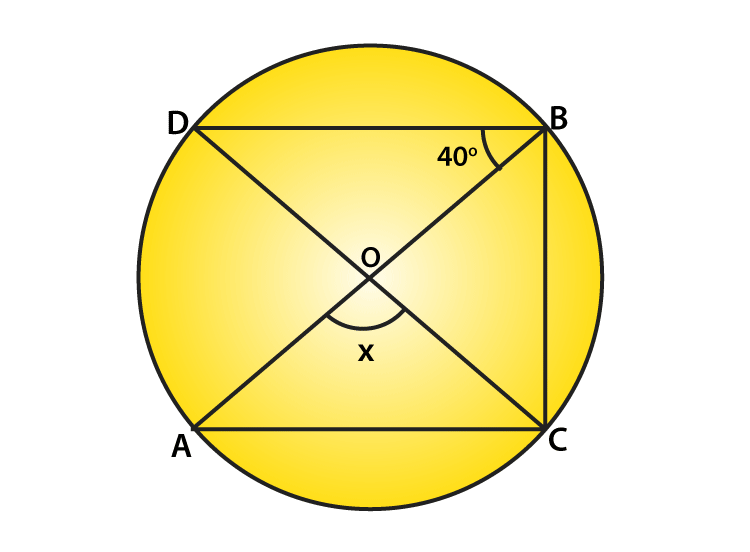Solution:

∠DBO = 400 (Given)

Form figure:

∠DBC = 900 [Angle in a semicircle]

∠DBO + ∠OBC = 900

400+∠OBC=900

or ∠OBC=900−400=500

Again, By degree measure theorem: ∠AOC = 2∠OBC

or x = 2×500=1000

(ix)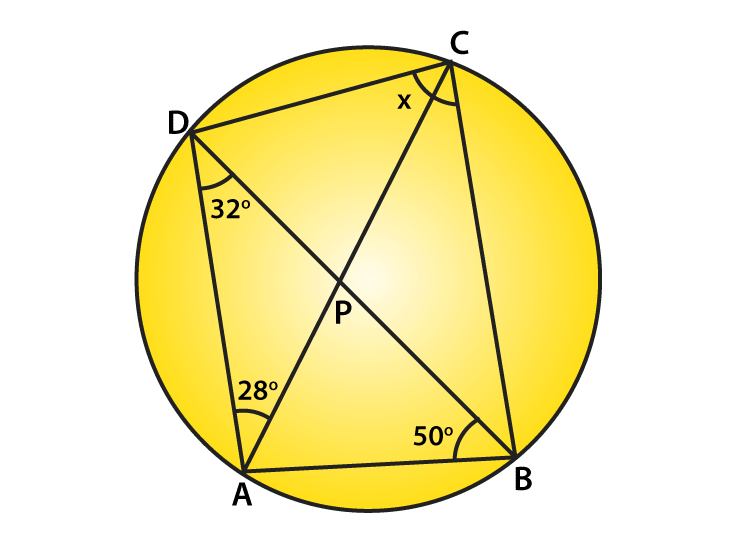Solution:

From figure:

In ΔDAB:

Angle sum property: ∠ADB + ∠DAB + ∠ABD = 1800

By substituting the given values, we get

320 + ∠DAB + 500 = 1800

∠DAB=1800−320−500

∠DAB = 980

Now,

∠DAB+∠DCB=1800 [Opposite angles of cyclic quadrilateral, their sum = 180 degrees]

980+x=1800

or x = 1800−980=820

The value of x is 82 degrees.

(x)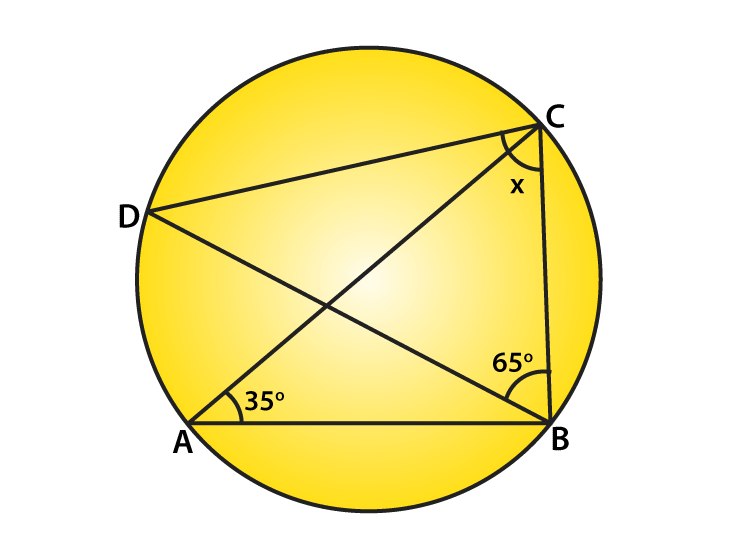Solution:

∠BAC = 350 and ∠DBC = 650

From figure:

∠BDC = ∠BAC = 350 [Angle in same segment]

In ΔBCD:

Angle sum property, we have

∠BDC + ∠BCD + ∠DBC = 1800

350 + x + 650 = 1800

or x = 1800 – 350 – 650 = 800

(xi)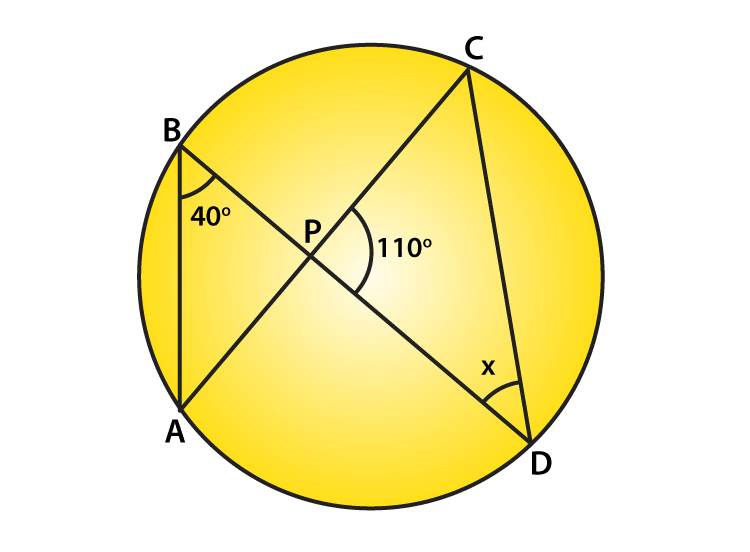Solution:

∠ABD = 400, ∠CPD = 1100 (Given)

Form figure:

∠ACD = ∠ABD = 400 [Angle in same segment]

In ΔPCD,

Angle sum property: ∠PCD+∠CPO+∠PDC=1800

400 + 1100 + x = 1800

x=1800−1500 =300

The value of x is 30 degrees.

(xii)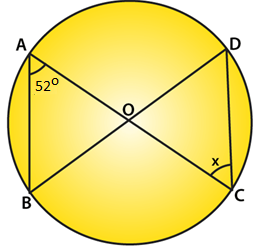Solution:

∠BAC = 520 (Given)

From figure:

∠BDC = ∠BAC = 520 [Angle in same segment]

Since OD = OC (radii), then ∠ODC = ∠OCD [Opposite angle to equal radii]

So, x = 520

Question 5: O is the circumcentre of the triangle ABC and OD is perpendicular on BC. Prove that ∠BOD = ∠A.

Solution: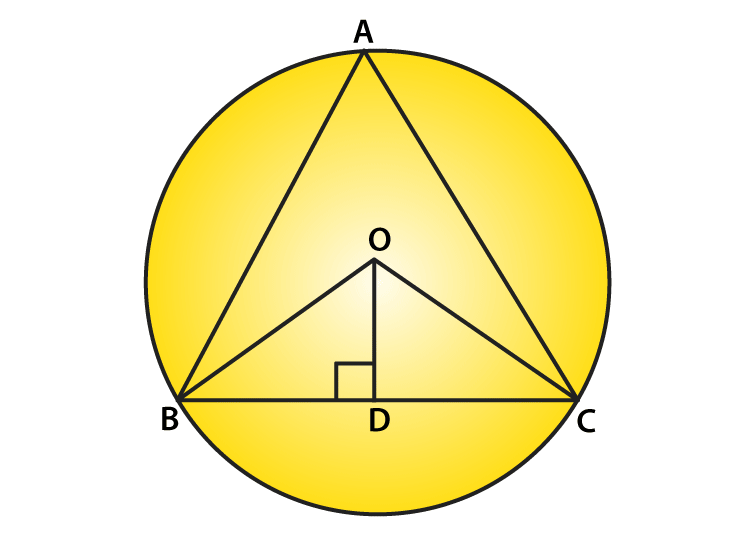In ΔOBD and ΔOCD:

∠ODB = ∠ODC [Each 900]

OD = OD [Common]

Therefore, By RHS Condition

ΔOBD ≅ ΔOCD

So, ∠BOD = ∠COD…..(i)[By CPCT]

Again,

By degree measure theorem: ∠BOC = 2∠BAC

2∠BOD = 2∠BAC [Using(i)]

∠BOD = ∠BAC

Hence proved.

Question 6: In figure, O is the centre of the circle, BO is the bisector of ∠ABC. Show that AB = AC.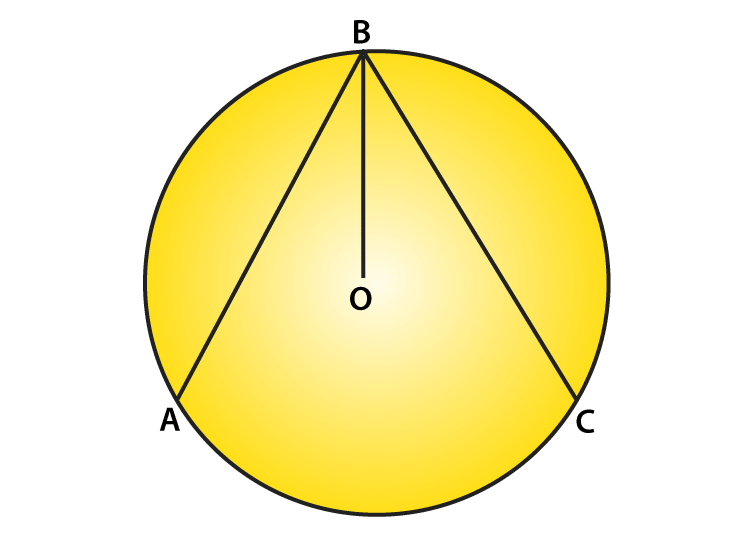Solution:

Since, BO is the bisector of ∠ABC, then,

∠ABO = ∠CBO …..(i)

From figure:

Radius of circle = OB = OA = OB = OC

∠OAB = ∠OCB …..(ii) [opposite angles to equal sides]

∠ABO = ∠DAB …..(iii) [opposite angles to equal sides]

From equations (i), (ii) and (iii), we get

∠OAB = ∠OCB …..(iv)

In ΔOAB and ΔOCB:

∠OAB = ∠OCB [From (iv)]

OB = OB [Common]

∠OBA = ∠OBC [Given]

Then, By AAS condition : ΔOAB ≅ ΔOCB

So, AB = BC [By CPCT]

Question 7: In figure, O is the centre of the circle, then prove that ∠x = ∠y + ∠z.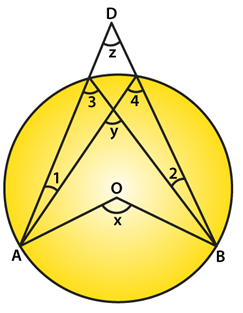Solution:

From the figure:

∠3 = ∠4 ….(i) [Angles in same segment]

∠x = 2∠3 [By degree measure theorem]

∠x = ∠3 + ∠3

∠x = ∠3 + ∠4 (Using (i) ) …..(ii)

Again, ∠y = ∠3 + ∠1 [By exterior angle property]

or ∠3 = ∠y − ∠1 …..(iii)

∠4 = ∠z + ∠1 …. (iv) [By exterior angle property]

Now, from equations (ii) , (iii) and (iv), we get

∠x = ∠y − ∠1 + ∠z + ∠1

or ∠x = ∠y + ∠z + ∠1 − ∠1

or x = ∠y + ∠z

Hence proved.

## RD Sharma Solutions for Class 9 Maths Chapter 16 Circles Exercise 16.4

Class 9 Maths Chapter 16 Circles Exercise 16.4 is based on the topic – Arcs and angles subtended by them. Students can practice more Maths concepts using RD Sharma solutions and clear their doubts.